# Phragmén-Lindelöf theorem

A generalization of the maximum-modulus principle for analytic functions to the case of functions that are given a priori as unbounded; it was first given in its simplest form by E. Phragmén and E. Lindelöf . Letbe a regular analytic function of a complex variablein a domainof the planewith boundary. One says thatdoes not exceed a numberin modulus at a boundary point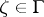if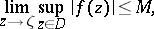that is, for everythere is a disc(depending onand) with centresuch thatfor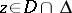. The main content of the result of Phragmén and Lindelöf, in a somewhat modernized form, consists in the following two propositions, which are successive extensions of the maximum-modulus principle.

1) If the regular analytic functionexceedsin modulus nowhere on, then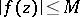everywhere in. This proposition is sometimes called the Phragmén–Lindelöf principle. It extends the maximum-modulus principle to functions about the behaviour of which on the boundary only partial information is available.

2) Suppose that the regular analytic functiondoes not exceedin modulus at any point ofnot belonging to some set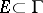. Suppose also that there is a functionwith the following properties: a)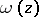is regular in; b)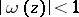in; c)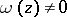in; and d) for everythe function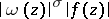does not exceedin modulus at any point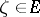. Under these conditionseverywhere in.

The Phragmén–Lindelöf theorem has received numerous applications, also often called Phragmén–Lindelöf theorems, and associated with a concrete form of,and(see , in particular the generalization given in ). In applicationsmost often consists of the single point. For example, suppose thatis regular in the angular domain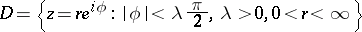(*)

and does not exceedin modulus on the sides of the angle. Then the following alternative holds: Eithereverywhere in, or the maximum modulusincreases faster thanasfor any,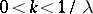. This theorem is obtained from propositions 1 and 2 for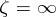,, where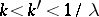.

The statements of 1 and 2 remain valid for a holomorphic function,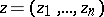, given in a domainof the complex space,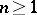. Many papers have been devoted to obtaining results of the type of the Phragmén–Lindelöf theorem for the solutions of partial differential equations and systems of equations of elliptic type. Propositions 1 and 2 remain true for a subharmonic function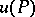defined in a domainof a Euclidean space,, or,, provided thatis replaced byand the function,, is assumed to be logarithmically subharmonic (cf. Logarithmically-subharmonic function) in(see , ). For example, suppose that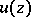is a subharmonic function in the angular domain (*) and does not exceedin modulus on the sides of the angle. Then the following alternative holds: Eithereverywhere in, or the maximumincreases faster than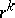for every,. There are also similar results for solutions of other elliptic equations. They may be called "weak" theorems of Phragmén–Lindelöf type, in the sense that, on account of their weak restriction only on the function itself on the boundary, one obtains a fairly weak assertion about its growth.

In other results, which may be called "strong" theorems of Phragmén–Lindelöf type, on account of the restriction on the function itself and its normal derivative on the boundary, one obtains a stronger assertion about its growth. An example is the following statement for the cylindrical domainin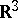. Suppose thatis a harmonic function in the cylinderand on its lateral surface, with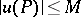and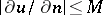on. Then eithereverywhere in, or the maximum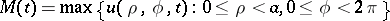increases, as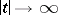, faster than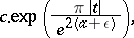for any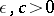(see ).

How to Cite This Entry:
Phragmén-Lindelöf theorem. Encyclopedia of Mathematics. URL: http://encyclopediaofmath.org/index.php?title=Phragm%C3%A9n-Lindel%C3%B6f_theorem&oldid=23452
This article was adapted from an original article by E.D. Solomentsev (originator), which appeared in Encyclopedia of Mathematics - ISBN 1402006098. See original article